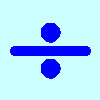# Search by Topic

Filter by: Content type:
Age range:
Challenge level:

### There are 4 results

Broad Topics > NC > NC-ProblemSolvingMD-R1-Y5### Division Rules

##### Age 7 to 11 Challenge Level:

This challenge encourages you to explore dividing a three-digit number by a single-digit number.### Curious Number

##### Age 7 to 11 Challenge Level:

Can you order the digits from 1-3 to make a number which is divisible by 3 so when the last digit is removed it becomes a 2-figure number divisible by 2, and so on?### Odd Squares

##### Age 7 to 11 Challenge Level:

Think of a number, square it and subtract your starting number. Is the number you’re left with odd or even? How do the images help to explain this?### Cubes Within Cubes

##### Age 7 to 14 Challenge Level:

We start with one yellow cube and build around it to make a 3x3x3 cube with red cubes. Then we build around that red cube with blue cubes and so on. How many cubes of each colour have we used?